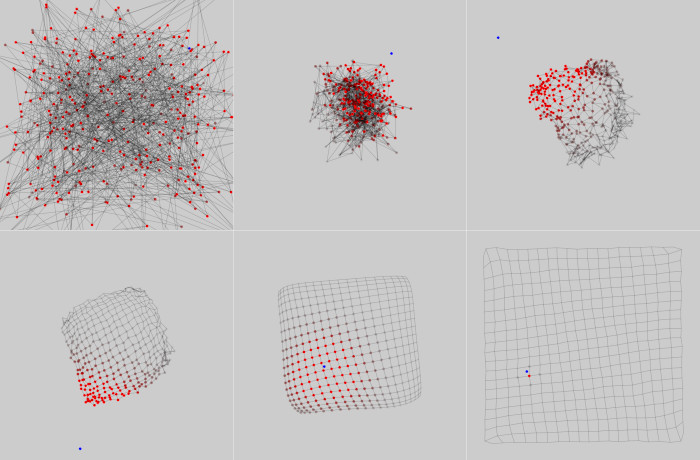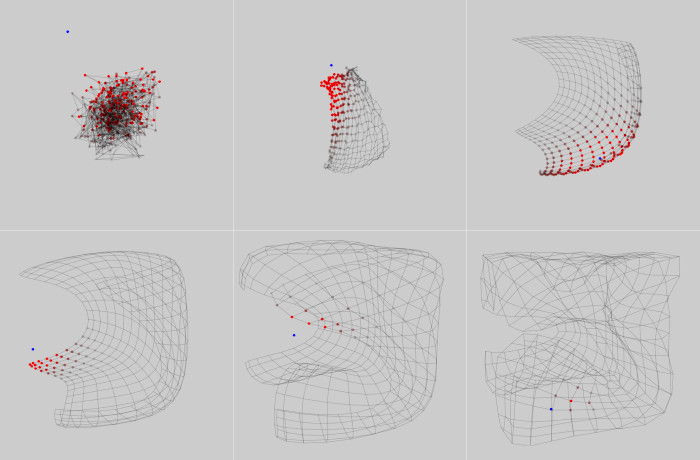active: late 2006

# Self-Organizing Maps

Explorations in 2D and 3D

Self-organizing maps (SOMs) perform a kind of topographically-constrained data compression. Ignoring the topographic constraint, they are essentially vector quantizers, where each data point is assigned to one of a finite number of units based on a distance metric. The topographic aspect adds an additional force which ensures "nearby" units represent nearby point in data space.

Put another way, they map a vector from some input space (usually of high dimensionality) onto a vector in some output space (usually 2-dimensional) while maintaining topographic relationships (vectors that are similar in the input space correspond to vectors that are similar in the output space). The interesting part is learning this mapping, which is usually performed unsupervised (i.e. just from exposure to lots of data).

To learn more about them, I implemented Kohonen's basic SOM algorithm. I wanted to gain some intuition concerning how they adapt over time, so I set up a 3D visualization which shows the map moving through the input space as data is acquired (in an incremental learning mode, not batch). Below are some results from training a map on a uniform sampling of both a 2D square and a 3D cube. The blue dot is the sample input point. The translucent red dots represent the winning node's neighborhood, which diminishes over time. Notice how in each case the maps try to fill the input space as well as possible (but note that Kohonen's original SOM does not achieve optimal spacing, i.e. the density of the SOM neuron centers does not match the probability density of the data distribution).SOM learning to represent a uniform 2D square distributionSOM learning to represent a uniform 3D cube distribution